# Resonance

## AC Electric Circuits

• #### Question 1

The Q factor of a series inductive circuit is given by the following equation:

 Q = XL Rseries

Likewise, we know that inductive reactance may be found by the following equation:

 XL = 2 πf L

We also know that the resonant frequency of a series LC circuit is given by this equation:

fr = 1

 2 π √ LC

Through algebraic substitution, write an equation that gives the Q factor of a series resonant LC circuit exclusively in terms of L, C, and R, without reference to reactance (X) or frequency (f).

• #### Question 2

 Don’t just sit there! Build something!!

Learning to mathematically analyze circuits requires much study and practice. Typically, students practice by working through lots of sample problems and checking their answers against those provided by the textbook or the instructor. While this is good, there is a much better way.

You will learn much more by actually building and analyzing real circuits, letting your test equipment provide the “answers” instead of a book or another person. For successful circuit-building exercises, follow these steps:

1. Carefully measure and record all component values prior to circuit construction.
2. Draw the schematic diagram for the circuit to be analyzed.
3. Carefully build this circuit on a breadboard or other convenient medium.
4. Check the accuracy of the circuit’s construction, following each wire to each connection point, and verifying these elements one-by-one on the diagram.
5. Mathematically analyze the circuit, solving for all voltage and current values.
6. Carefully measure all voltages and currents, to verify the accuracy of your analysis.
7. If there are any substantial errors (greater than a few percent), carefully check your circuit’s construction against the diagram, then carefully re-calculate the values and re-measure.

For AC circuits where inductive and capacitive reactances (impedances) are a significant element in the calculations, I recommend high quality (high-Q) inductors and capacitors, and powering your circuit with low frequency voltage (power-line frequency works well) to minimize parasitic effects. If you are on a restricted budget, I have found that inexpensive electronic musical keyboards serve well as “function generators” for producing a wide range of audio-frequency AC signals. Be sure to choose a keyboard “voice” that closely mimics a sine wave (the “panflute” voice is typically good), if sinusoidal waveforms are an important assumption in your calculations.

As usual, avoid very high and very low resistor values, to avoid measurement errors caused by meter “loading”. I recommend resistor values between 1 kΩ and 100 kΩ.

One way you can save time and reduce the possibility of error is to begin with a very simple circuit and incrementally add components to increase its complexity after each analysis, rather than building a whole new circuit for each practice problem. Another time-saving technique is to re-use the same components in a variety of different circuit configurations. This way, you won’t have to measure any component’s value more than once.

• #### Question 3

Not only do reactive components unavoidably possess some parasitic (“stray”) resistance, but they also exhibit parasitic reactance of the opposite kind. For instance, inductors are bound to have a small amount of capacitance built-in, and capacitors are bound to have a small amount of inductance built-in. These effects are not intentional, but they exist anyway.

Describe how a small amount of capacitance comes to exist within an inductor, and how a small amount of inductance comes to exist within a capacitor. Explain what it is about the construction of these two reactive components that allows the existence of “opposite” characteristics.

• #### Question 4

Calculate the resonant frequency of this parallel LC circuit, and qualitatively describe its total impedance (Ztotal) when operating at resonance:• #### Question 5

If a metal bar is struck against a hard surface, the bar will “ring” with a characteristic frequency. This is the fundamental principle upon which tuning forks work: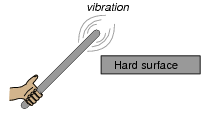The ability of any physical object to “ring” like this after being struck is dependent upon two complementary properties: mass and elasticity. An object must possess both mass and a certain amount of “springiness” in order to physically resonate.

Describe what would happen to the resonant frequency of a metal bar if it were made of a more elastic (less “stiff”) metal? What would happen to the resonant frequency if an extra amount of mass were added to the end being struck?

• #### Question 6

This simple electric circuit is capable of resonance, whereby voltage and current oscillate at a frequency characteristic to the circuit: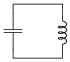In a mechanical resonant system - such as a tuning fork, a bell, or a guitar string - resonance occurs because the complementary properties of mass and elasticity exchange energy back and forth between each other in kinetic and potential forms, respectively. Explain how energy is stored and transferred back and forth between the capacitor and inductor in the resonant circuit shown in the illustration, and identify which of these components stores energy in kinetic form, and which stores energy in potential form.

• #### Question 7

Resonant electric circuits are analogous to resonant mechanical systems. They both oscillate, and their oscillation is founded on an exchange of energy between two different forms.

Mechanical engineers studying vibrations in machinery sometimes use capacitors and inductors to model the physical characteristics of mechanical systems. Specifically, capacitors model elasticity, while inductors model mass.

Explain what mechanical quantities in a resonant system are analogous to voltage and current in a resonant circuit.

• #### Question 8

If an oscilloscope is set up for “single-sweep” triggering and connected to a DC-excited resonant circuit such as the one shown in the following schematic, the resulting oscillation will last just a short time (after momentarily pressing and releasing the pushbutton switch):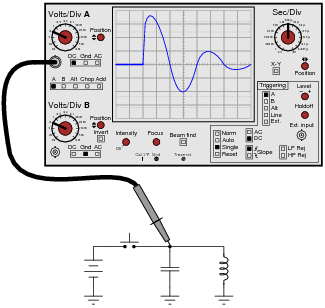Explain why the oscillations die out, rather than go on forever. Hint: the answer is fundamentally the same as why a swinging pendulum eventually comes to a stop.

• #### Question 9

How may the resonant frequency of this tank circuit be increased?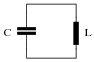• #### Question 10

Very interesting things happen to resonant systems when they are “excited” by external sources of oscillation. For example, a pendulum is a simple example of a mechanically resonant system, and we all know from experience with swings in elementary school that we can make a pendulum achieve very high amplitudes of oscillation if we “oscillate” our legs at just the right times to match the swing’s natural (resonant) frequency.

Identify an example of a mechanically resonant system that is “excited” by an external source of oscillations near its resonant frequency. Hint: research the word “resonance” in engineering textbooks, and you are sure to read about some dramatic examples of resonance in action.

• #### Question 11

If a capacitor and an inductor are connected in series, and energized by an AC voltage source with such a frequency that the reactances of each component are 125 Ω and 170 Ω, respectively, what is the total impedance of the series combination?

• #### Question 12

Calculate all voltage drops and current in this LC circuit at each of the given frequencies:Frequency VL VC Itotal 50 Hz 60 Hz 70 Hz 80 Hz 90 Hz 100 Hz

Also, calculate the resonant frequency of this circuit.

• #### Question 13

Suppose we were to build a series “LC” circuit and connect it to a function generator, where we could vary the frequency of the AC voltage powering it:Calculate the amount of current in the circuit, given the following figures:

Power supply voltage = 3 volts RMS
Power supply frequency = 100 Hz
Capacitor = 4.7 μF
Inductor = 100 mH

Then, describe what happens to the circuit current as the frequency is gradually increased.

• #### Question 14

Calculate the power supply frequency at which the reactances of a 33 μF and a 75 mH inductor are exactly equal to each other. Derive a mathematical equation from the individual reactance equations $$(X_{L}=2 \pi fL\ and\ {X}_{C}=\frac{1}{2 \pi fC}$$, solving for frequency (f) in terms of L and C in this condition.

Calculate the total impedance of these two components, if they were connected in series, at that frequency.

• #### Question 15

Calculate all voltages and currents in this circuit, at a power supply frequency near resonance: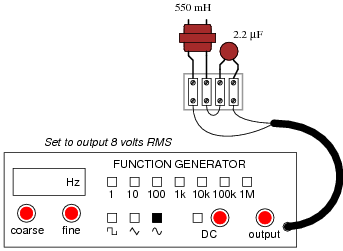Based on your calculations, what general predictions can you make about series-resonant circuits, in terms of their total impedance, their total current, and their individual component voltage drops?

• #### Question 16

Calculate all voltages and currents in this circuit, at a power supply frequency near resonance: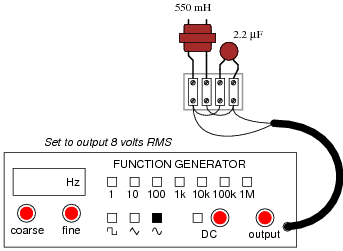Based on your calculations, what general predictions can you make about parallel-resonant circuits, in terms of their total impedance, their total current, and their individual component currents?

• #### Question 17

Does a series LC circuit “appear” capacitive or inductive (from the perspective of the AC source powering it) when the source frequency is greater than the circuit’s resonant frequency? What about a parallel resonant circuit? In each case, explain why.

• #### Question 18

A paradoxical property of resonant circuits is that they have the ability to produce quantities of voltage or current (in series and parallel circuits, respectively) exceeding that output by the power source itself. This is due to the cancellation of inductive and capacitive reactances at resonance.

Not all resonant circuits are equally effective in this regard. One way to quantify the performance of resonant circuits is to assign them a quality factor, or Q rating. This rating is very similar to the one given inductors as a measure of their reactive “purity.”

Suppose we have a resonant circuit operating at its resonant frequency. How may we calculate the Q of this operating circuit, based on empirical measurements of voltage or current? There are two answers to this question: one for series circuits and one for parallel circuits.

• #### Question 19

Shown here are two frequency response plots (known as Bode plots) for a pair of series resonant circuits. Each circuit has the same inductance and capacitance values, but different resistance values. The “output” is voltage measured across the resistor of each circuit: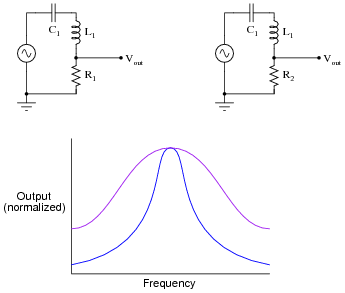Which one of these plots represents the response of the circuit with the greatest Q, or quality factor?

• #### Question 20

The Q, or quality factor, of an inductor circuit is defined by the following equation, where Xs is the series inductive reactance and Rs is the series resistance:

 Q = Xs Rs

We also know that we may convert between series and parallel equivalent AC networks with the following conversion equations:

 Rs Rp = Z2 Xs Xp = Z2Series and parallel LR networks, if truly equivalent, should share the same Q factor as well as sharing the same impedance. Develop an equation that solves for the Q factor of a parallel LR circuit.

• #### Question 21

There is a direct, mathematical relationship between bandwidth, resonant frequency, and Q in a resonant filter circuit, but imagine for a moment that you forgot exactly what that formula was. You think it must be one of these two, but you are not sure which:

 Bandwidth = Q fr (or possibly) Bandwidth = fr Q

Based on your conceptual knowledge of how a circuit’s quality factor affects its frequency response, determine which of these formulae must be incorrect. In other words, demonstrate which of these must be correct rather than simply looking up the correct formula in a reference book.

• #### Question 22

Suppose you have a 110 mH inductor, and wish to combine it with a capacitor to form a band-stop filter with a “notch” frequency of 1 kHz. Draw a schematic diagram showing what the circuit would look like (complete with input and output terminals) and calculate the necessary capacitor size to do this, showing the equation you used to solve for this value. Also, calculate the bandwidth of this notch filter, assuming the inductor has an internal resistance of 20 ohms, and that there is negligible resistance in the rest of the circuit.

• #### Question 23

Shown here are two frequency response plots (known as Bode plots) for a pair of series resonant circuits with the same resonant frequency. The “output” is voltage measured across the resistor of each circuit: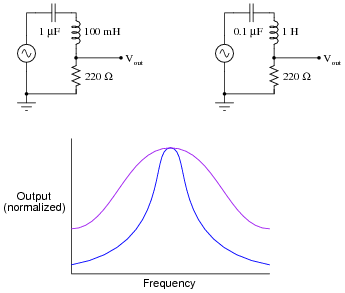Determine which plot is associated with which circuit, and explain your answer.

• #### Question 24

Given the unavoidable presence of parasitic inductance and/or capacitance in any electronic component, what does this mean in terms of resonance for single components in AC circuits?

• #### Question 25

A capacitor has been connected in parallel with the solenoid coil to minimize arcing of the switch contacts when opened: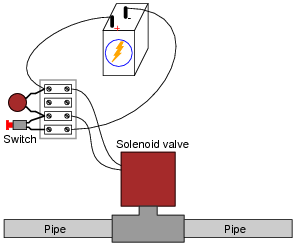The only problem with this solution is, resonance between the capacitor and the solenoid coil’s inductance is causing an oscillating voltage (commonly known as ringing) to appear across the terminals of each. This high-frequency “ringing” is generating bursts of radio interference whenever the switch contacts open. Radio interference is not good.

You know that the underlying cause of this “ringing” is resonance, yet you cannot simply remove the capacitor from the circuit because you know that will result in decreased operating life for the switch contacts, as the solenoid’s inductive “kickback” will cause excessive arcing. How do you overcome this problem without creating another problem?